# Addition & Product of 2 Graphs Rank and Nullity of a Graph

• Last Updated : 22 Jun, 2021

Introduction :
A Graph G consists of vertices & edges. The edges are lines or arcs that connect any two nodes in the graph, and the nodes are also known as vertices.

A simple Graph G = (V, E) consists of :

Attention reader! Don’t stop learning now.  Practice GATE exam well before the actual exam with the subject-wise and overall quizzes available in GATE Test Series Course.

Learn all GATE CS concepts with Free Live Classes on our youtube channel.

• V : Finite set of vertices
• E : Set of edges

Example –
In the diagram below, Graph G consists of :
V = { A, B, C, D}
E = { {A, C}, {C, B}, {A, D}}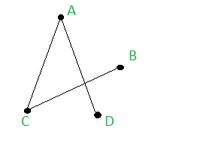If we have 2 graphs, G1 & G2 such that their vertices intersection is null ( V(G1)∩ V(G2) = ∅ ) , then the sum :
G1 + G2 is defined as the graph whose vertex set V(G1+G2) is V(G1) + V(G2) and the edge set consists of these edges, which are inG1 & in G2 & the edges contained by joining each vertex of G1 to each vertex of G2.
Example : The addition of 2 graphs shown G1 & G2 are :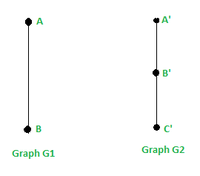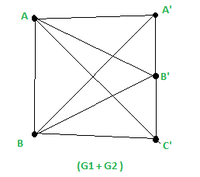Here : V(G1)∩ V(G2) = ∅

The already contained edges in G1 are, E(G1) : {{A, B}} and the vertices are : V(G1) = {A, B}
The already contained edges in G2 are,  E(G2) : {{A’, B’} ,{B’,C’} }and the vertices are : V(G2) = {A’, B’, C’}
So the graph , G1 + G2 will have
(i) vertices as : V(G1+G2 ) = V(G1) + V(G2) = { A, B. A’, B’, C’}
(ii) and E(G1 + G2 ) = E(G1) + E(G2) +  edges contained by joining each vertex of G1 to each vertex of G2 =
{ {A, B}, {A’, B’} ,{B’,C’} , {A,A’} , {A, B’}, {A, C’}, {B,A’} , {B, B’}, {B, C’}}

Product of 2 Graphs :
We define the product of 2 graphs, G1*G2, as (G1*G2)(V, E) such that :
(i) Vertices : V(G1*G2 ) = Cartesian product of V(G1) & V(G2) =  V(G1) * V(G2) and
(ii) Edges : Considering any 2 vertices in the set of vertices of the graph G1*G2 (i.e, the cartesian product of V(G1) & V(G2) )  , say A & V (Note : A & V are vertex that is a pair of 2 elements) such that  : A = (a1, a2) & V = (v1,v2) , then {A, V} is an edge in graph G1*G2 if one of the following is satisfied –
(i) a1 = v1 ( the first element of the pair is same) and a2 is adjacent to v2
(ii) a2 = v2 ( the second element of the pair is same) and a1 is adjacent to v1

Example : Consider 2 Graphs, G1 & G2 such that :
V(G1) = {A, B}
E(G1) = { {A, B} }
V(G2) = {A’, B’, C’}
E(G2) ={ {A’, B’} ,{B’,C’} }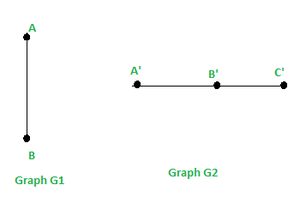Then Graph G1*G2 will have :
(i) Vertices : V(G1*G2 ) = cartesian product of V(G1) & V(G2) =  V(G1) * V(G2)  = { (A, A’) , (A, B’), (A, C’), (B, A’) , (B, B’), (B, C’) }
(ii) Edges : We need to check for every pair of vertex in G1 * G2 that it can form an edge / not . As we know, that if we have 2 vertices  A & V in (G1 * G2) such that  : A = (a1, a2) & V = (v1,v2) , then {A, V} is an edge in graph G1*G2 if one of the following is satisfied –
(i) a1 = v1 (first element of pair is same) AND a2 is adjacent to v2
(ii) a2 = v2 (second element of pair is same) AND a1 is adjacent to v1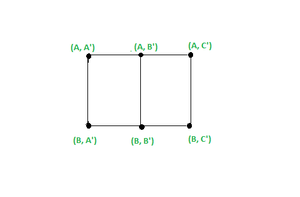Graph Product G1 * G2

We find that :
1.  { (A, A’) , (A, B’) } is an edge because the first element of the pair is same (A = A) and A’ is adjacent to B’ in G2
2.  { (A, C’) , (A, B’) } is an edge because the first element of the pair is same (A = A) and C’ is adjacent to B’ in G2
3.  { (B, A’) , (B, B’) } is an edge because the first element of the pair is same (B = B) and A’ is adjacent to B’ in G2
4.  { (B, C’) , (B, B’) } is an edge because the first element of the pair is same (A = A) and C’ is adjacent to B’ in G2
5.  { (A, A’) , (B, A’) } is an edge because the second element of the pair is same (A’ = A’) and A is adjacent to B in G1
6.  { (A, B’) , (B, B’) } is an edge because the second element of the pair is same (B’ = B’) and A is adjacent to B in G1
7.  { (A, C’) , (B, C’) } is an edge because the second element of the pair is same (C’ = C’) and A is adjacent to B in G1

So E(G1*G2) = {  { (A, A’) , (A, B’) } ,  { (A, C’) , (A, B’) } ,  { (B, A’) , (B, B’) } ,  { (B, C’) , (B, B’) } ,  { (A, A’) , (B, A’) } , { (A, B’) , (B, B’) } ,  { (A, C’) , (B, C’) } }

In this way we can find the addition & product of any 2 graphs.

Rank & Nullity of a Graph :
Let G(V,E) be a graph with n vertices & m edges and K Components.
i.e.;  |G(V)| = n & |G(E)| = m we define the rank P(G) & nullity μ(G) as follows :

```If G Is not connected :
P(G) = Rank of G = n - k
μ(G) = Nullity of G = m - n + k

If G Is connected :
P(G) = Rank of G = n - 1
μ(G) = Nullity of G = m - n + 1```

Example 1 :The Graph shown below is connected :Graph G

|G(V)| = n = 4 &
|G(E)| = m = 3
P(G) = Rank of G = n – 1 = 4 -1 = 3
μ(G) = Nullity of G = m – n + 1 = 3 – 4  + 1 = 0

Example 2 : The Graph shown below is not connected :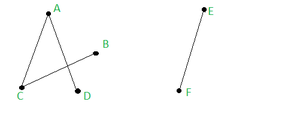|G(V)| = n = 6 &
|G(E)| = m = 4 &
No of components = k = 2
P(G) = Rank of G = n – k = 6 – 2 = 4
μ(G) = Nullity of G = m – n + k = 4 – 6  + 2 = 0

My Personal Notes arrow_drop_up